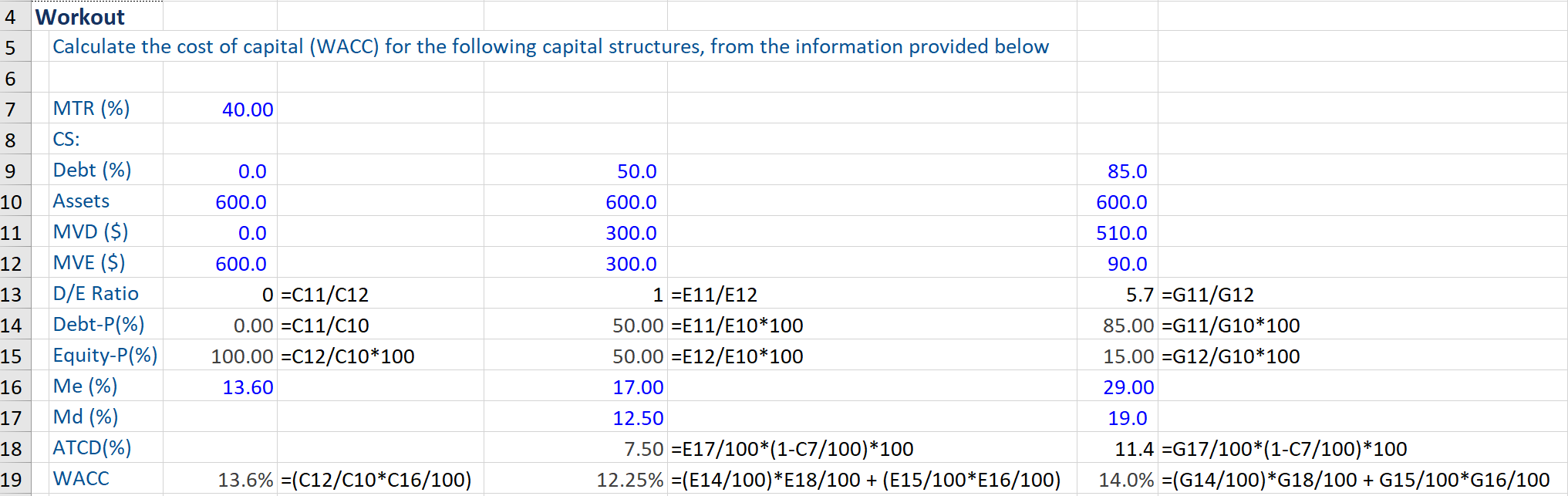## What is “Optimal Capital Structure?”

The capital structure of a company refers to the proportion of debt and equity it uses to finance its business (i.e. finance its operations, capital expenditures, acquisitions, etc.). Having stated this, the optimal capital structure refers to the best or right mix of debt and equity that minimizes a company’s cost of capital, more specifically, the weighted average cost of capital (WACC), while maximizing its market value (i.e. shareholder wealth). The lower the cost of capital, the higher will be the company’s market value.

The optimal capital structure of a company is impacted by WACC, cost of debt, and cost of equity. Cost of capital is one of the major considerations that companies must take into account while deciding on their optimal capital structure. For any worthwhile investment, the expected return on capital must be higher than the cost of capital.

## Key Learning Points

• In determining an optimal capital structure, a company needs to take into account several factors, and tradeoffs between debt and equity.
• Calculation of WACC involves calculating the marginal cost of each source of financing and taking a weighted average of the same.
• In practice, one cannot specifically pinpoint at which level of debt a company reaches the optimal capital structure.

## Optimal Capital Structure, factors, cost of capital, and trade-offs

To determine the company’s optimal capital structure, the company needs to take into account factors such as weighted average cost of capital, risk and expected return, business risk, industry averages, the potential cost of financial distress, company’s tax status, and application of financial models for this purpose.

Companies need to determine the capital structure that maximizes the company’s market value by minimizing their cost of capital. An optimal capital structure should result in the lowest WACC. The same is estimated by calculating the marginal cost of each source of financing used and thereafter taking a weighted average of the same. The formula for WACC (assuming that a company is using both debt and equity in its capital structure) is:

WACC (%) = MVD / (MVD + MVE) * Md(1-MTR) + MVE / (MVD + MVE) *Me

MVD = Market Value of Outstanding or Total Debt
MVE = Market Value of Total Equity
MVD + MVE = Total market value of the company’s combined debt and equity
MTR = Marginal tax rate of the company (%)
Md = Before-tax marginal cost of debt (%)
Me = Marginal Cost of Equity (%)
The After-tax cost of debt (ATCD) = Md (1-MTR). As interest is typically a tax-deductible expense, the WACC computation incorporates the after-tax cost of debt.

While deciding on the optimal capital structure, companies can issue either more debt or equity and here they face trade-offs. This is because while debt issuance has tax advantages and has a lower cost than equity, at higher levels of debt the default risk rises and investors will require a higher return. Therefore, companies need to identify the optimal mix of financing. The optimal capital structure involves using sufficient equity financing to mitigate the default risk associated with debt.

## Capital Structure, Scenarios, and WACC – Example

In practice, one cannot exactly pinpoint at which point or level of debt a company reaches the optimal capital structure and there is no specific debt/equity ratio that can be used as a guide to achieving such a capital structure. Therefore, companies attempt to have the desired proportion of debt and equity financing that they want to achieve and maintain i.e. the target capital structure.

Given below is an example of a company that wants to set up a subsidiary that would require assets worth \$600 million. It wants to have a capital structure (CS) that minimizes the WACC for this subsidiary. The company wants to assess the cost of capital i.e. WACC if it has a capital structure of 0% debt and 100% equity, 50% debt and 50% equity or 85% debt and 15% equity. Further, the company’s marginal tax rate is 40%.

Next, the cost of equity rises with additional levels of debt in the capital structure. When there is no debt, the marginal cost of equity is 13.6%, 17% at 50% debt, and 29% when there is 85% debt in the capital structure. The marginal cost of debt i.e. (before-tax marginal cost of debt) is 12.5% when there is 50% debt and 19% at 80% debt in the capital structure.

The cost of capital i.e. WACC is the lowest (12.25%) for a capital structure with 50% debt and 50% equity financing.